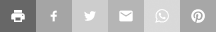The Physics
Hypertextbook
Opus in profectus

# Light is an electromagnetic disturbance# A Dynamical Theory of the Electromagnetic Field

## Part I. — Introduction (excerpt)

The general equations are next applied to the case of a magnetic disturbance propagated through a non-conducting field, and it is shown that the only disturbances which can be propagated are those which are transverse to the direction of propagation, and that the velocity of propagation is the velocity v, found from experiments such as those of Weber, which express the number of electrostatic units of electricity which are contained in one electromagnetic unit.

This velocity is so nearly that of light, that it seems we have strong reasons to conclude that light itself (including radiant heat, and other radiations if any) is an electromagnetic disturbance in the form of waves propagated through the electromagnetic field according to electromagnetic laws. If so, the agreement between the elasticity of the medium as calculated from the rapid alternations of luminous vibrations, and as found by the slow process of electrical experiments, shows how perfect and regular the elastic properties of the medium must be when not encumbered with any matter denser than air. If the same character of the elasticity is retained in dense transparent bodies, it appears that the square of the index of refraction is equal to the product of the specific dielectric capacity and the specific magnetic capacity. Conducting media are shown to absorb such radiations rapidly, and therefore to be generally opaque.

## Part VI. — Electromagnetic Theory of Light (excerpt)

By the electromagnetic experiments of MM. Weber and Kohlrausch,

v = 310,740,000 meters per second

is the number of electrostatic units in one electromagnetic unit of electricity, and this, according to our result, should be equal to the velocity of light in air or in a vacuum.

The velocity of light in air, by M. Fizeau's experiments, is

v = 314,858,000

according to the more accurate experiments of M. Foucault,

v = 298,000,000

The velocity of light in the space surrounding the Earth, deduced from the coefficient of aberration and the received value of the radius of the Earth's orbit, is

v = 308,000,000

Hence the velocity of light deduce from experiment agrees sufficiently well with the value of v deduced from the only set of experiments we yet possess. The value of v was determined by measuring the electromotive force with which a condenser of known capacity was charged, and then discharging the condenser through a galvanometer, so as to measure the quantity of electricity in it in electromagnetic measure. The only use made of light in the experiment was to see the instruments. The value of v found by M. Foucault was obtained by determining the angle through which a revolving mirror is turned, while the light reflected from it went and returned along a measured course. No use whatever was made of electricity or magnetism.

The agreement of the results seem to show that light and magnetism are affections of the same substance, and that light is an electromagnetic disturbance propagated through the field according to electromagnetic laws.